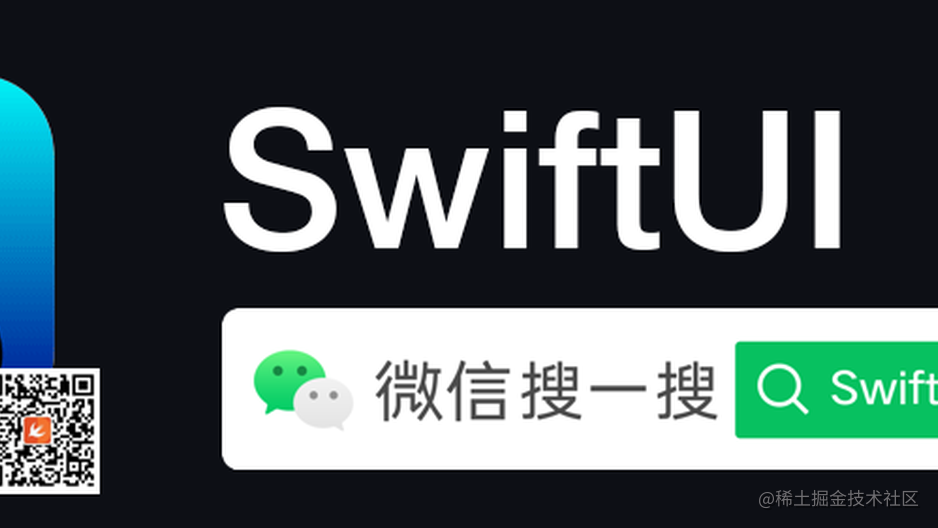父级视图想要什么？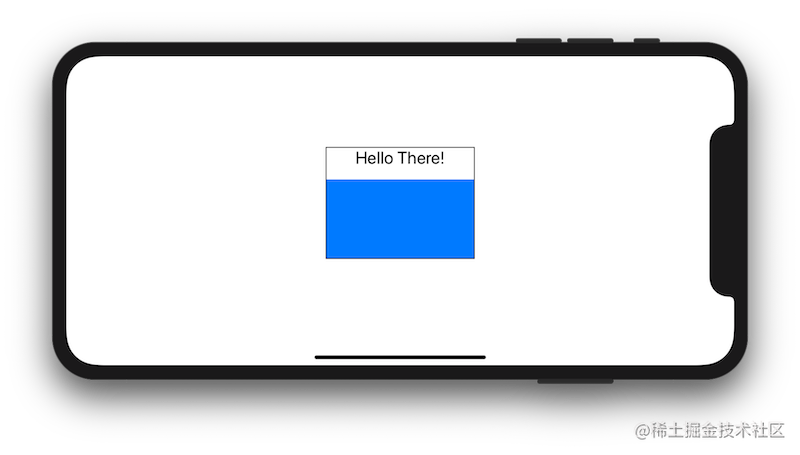struct ContentView : View {
var body: some View {

VStack {

Text("Hello There!")
MyRectangle()

}.frame(width: 150, height: 100).border(Color.black)

}
}

struct MyRectangle: View {
var body: some View {
Rectangle().fill(Color.blue)
}
}复制代码

子视图做了什么？

A container view that defines its content as a function of its own size and coordinate space.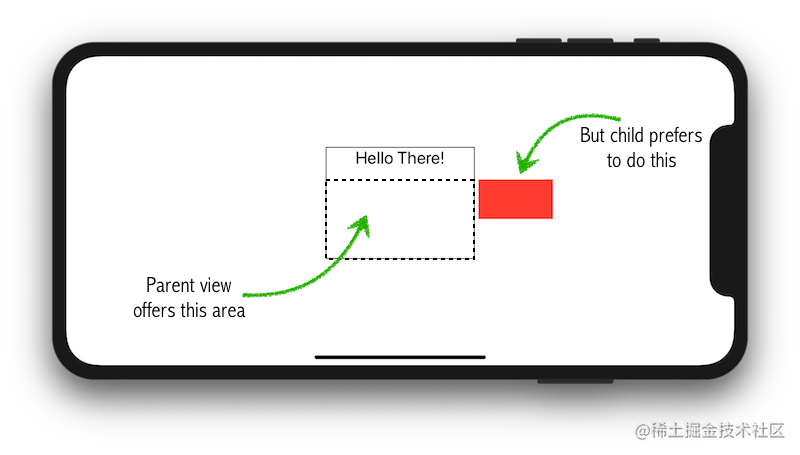struct ContentView : View {
var body: some View {

VStack {

Text("Hello There!")
MyRectangle()

}.frame(width: 150, height: 100).border(Color.black)

}
}

struct MyRectangle: View {
var body: some View {
Rectangle()
.path(in: CGRect(x: geometry.size.width + 5,
y: 0,
width: geometry.size.width / 2.0,
height: geometry.size.height / 2.0))
.fill(Color.blue)

}
}
}复制代码

GeometryProxy

public var size: CGSize { get }
public var safeAreaInsets: EdgeInsets { get }
public func frame(in coordinateSpace: CoordinateSpace) -> CGRect
public subscript<T>(anchor: Anchor<T>) -> T where T : Equatable { get }复制代码

GeometryProxy 把 safeAreaInsets 也暴露给了我们。

frame 方法暴露给我们父级视图建议区域的矩形，可以借助传入 .local, .global, .named() 来获取不同的坐标空间。本地和全局坐标空间不用多解释，.named()用来获取一个被命名的坐标空间。我们可以通过名称来获取其他视图的坐标空间。Inspecting the View Tree 这篇文章介绍了具体的使用方法。

吸收另一个视图的 Geometry

GeometryReader 功能已经非常强大，但如果它被结合 .background() 或者 .overlay() modifier 来使用，功能就会更加强大。

.overlay modifier 的道理一样，只不过它不是给被修改的视图绘制背景，而是叠在前面绘制。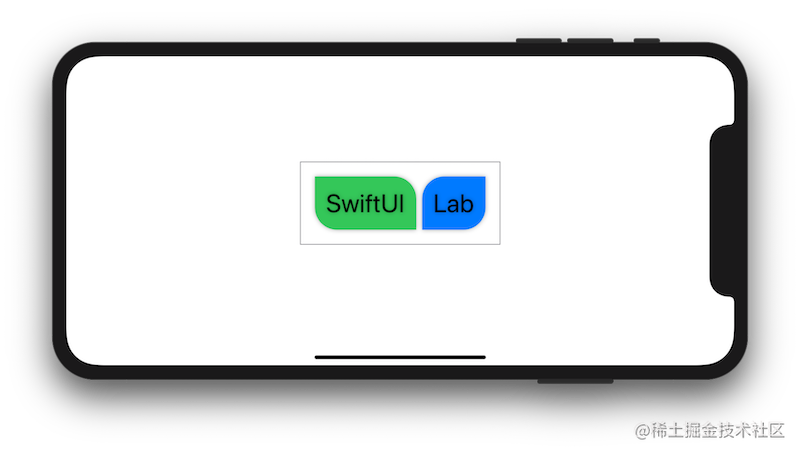struct ContentView : View {
var body: some View {

HStack {

Text("SwiftUI")
.background(RoundedCorners(color: .green, tr: 30, bl: 30))

Text("Lab")
.background(RoundedCorners(color: .blue, tl: 30, br: 30))

}
}

struct RoundedCorners: View {
var color: Color = .black
var tl: CGFloat = 0.0
var tr: CGFloat = 0.0
var bl: CGFloat = 0.0
var br: CGFloat = 0.0

var body: some View {
Path { path in

let w = geometry.size.width
let h = geometry.size.height

// 确保圆角半径不会超出界限
let tr = min(min(self.tr, h/2), w/2)
let tl = min(min(self.tl, h/2), w/2)
let bl = min(min(self.bl, h/2), w/2)
let br = min(min(self.br, h/2), w/2)

path.move(to: CGPoint(x: w / 2.0, y: 0))
path.addLine(to: CGPoint(x: w - tr, y: 0))
path.addArc(center: CGPoint(x: w - tr, y: tr), radius: tr, startAngle: Angle(degrees: -90), endAngle: Angle(degrees: 0), clockwise: false)
path.addLine(to: CGPoint(x: w, y: h - br))
path.addArc(center: CGPoint(x: w - br, y: h - br), radius: br, startAngle: Angle(degrees: 0), endAngle: Angle(degrees: 90), clockwise: false)
path.addArc(center: CGPoint(x: bl, y: h - bl), radius: bl, startAngle: Angle(degrees: 90), endAngle: Angle(degrees: 180), clockwise: false)
path.addArc(center: CGPoint(x: tl, y: tl), radius: tl, startAngle: Angle(degrees: 180), endAngle: Angle(degrees: 270), clockwise: false)
}
.fill(self.color)
}
}
}复制代码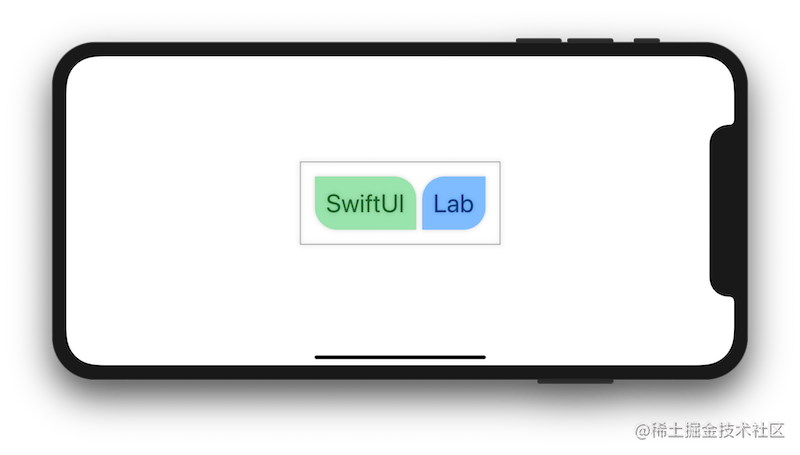Text("SwiftUI")
.overlay(RoundedCorners(color: .green, tr: 30, bl: 30).opacity(0.5))
Text("Lab")
.overlay(RoundedCorners(color: .blue, tl: 30, br: 30).opacity(0.5))复制代码

总结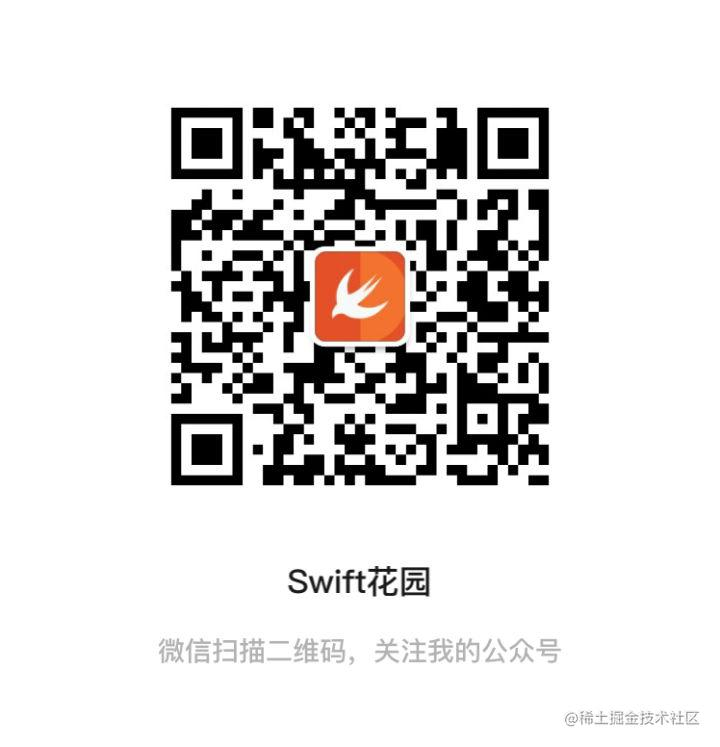iOS